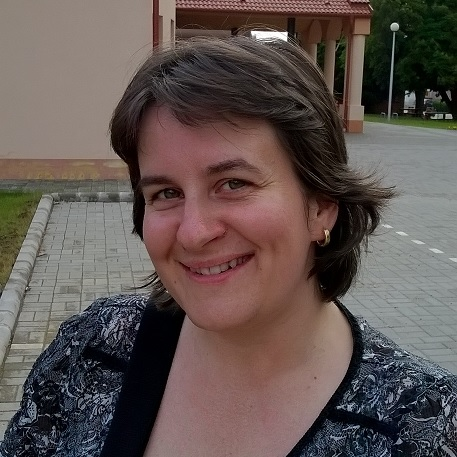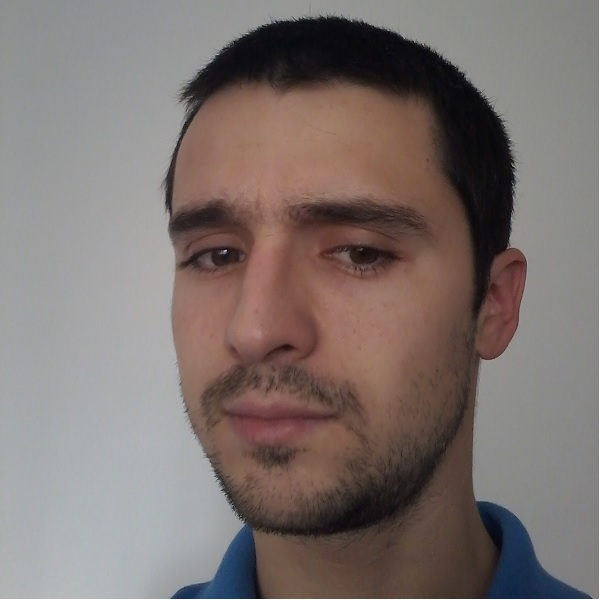# IT.I1.CALCULUS.0.ExCalculus I. Practical Exercises

With this course, you get a basic tool for problem-solving in applied mathematics.
False
registered False
in_cart False
is_course_full False

invitation_only False
can_enroll True

Calculus is the mathematical tool used to analyze changes in physical qunatities. It was developed int he seventeenth century to study four major classes of scientific and mathematical problems of the time:

• Find the tangent to a curve at a point.
• Find the length of a curve, the area of a region, and the volume of a solid.
• Find the maximum and minimum value of a quantity – for example, the maximum and minimum distances of a planet from the sun, or the maximum range attainable for a projectile by varying its angle of fire.
• Given a formula for the distance traveled by a body in any specified amount of time, find the velocity and acceleration of the body at any instant. Conversely, given a formula that specifies the acceleration or velocity at any instant, find the distance traveled by the body in a specified period of time.
• These problems were attacked by the greatest minds of the seventeeth century, culminating in the crowing achievements of Gottfried Wilhelm Leibniz and Isaac Newton – the creation of calculus.

-

## Course Staff### Dr. Zita Czapné Makó

assistant professor### Tamás Szakács

assistant lecturer

1. Course Number

IT.I1.CALCULUS.0.Ex
2. Classes Start

Nov 19, 2015
3. Classes End

Jan 20, 2016
4. Estimated Effort

25:00
Enroll